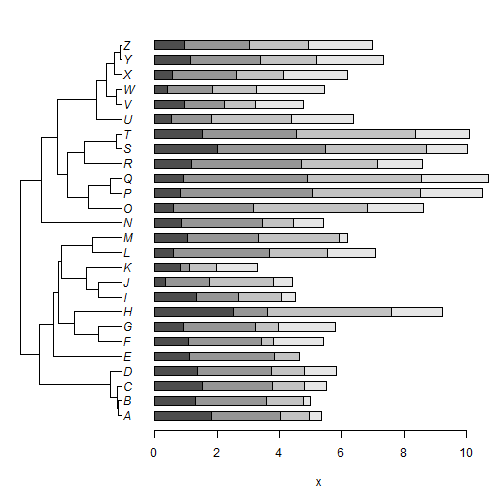## Wednesday, October 18, 2017

### `plotTree.barplot` in an array or with a custom layout

I just pushed a very small update to the phytools function `plotTree.barplot`.

The update allows for the optional argument `add`, which, when set to `TRUE`, will plot the tree & barplot in the next two open figures - rather than opening a new split figure using `par(mfrow=c(1,2))`.

Normally, we don't want this - because it will cause our tree & barplot to be plotted sequentially (to the same device) rather than side-by-side. E.g.:

``````library(phytools)
x<-fastBM(tree<-pbtree(n=26,tip.label=LETTERS,scale=1),a=2)
``````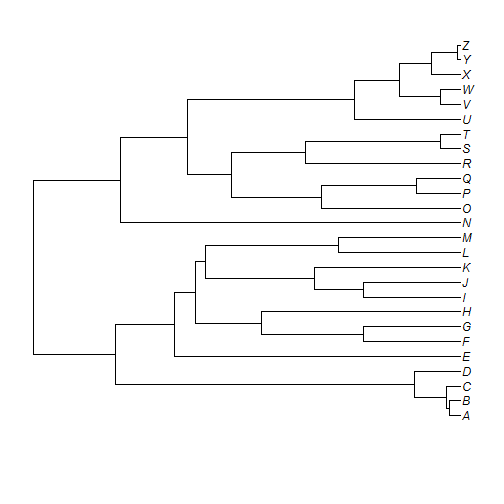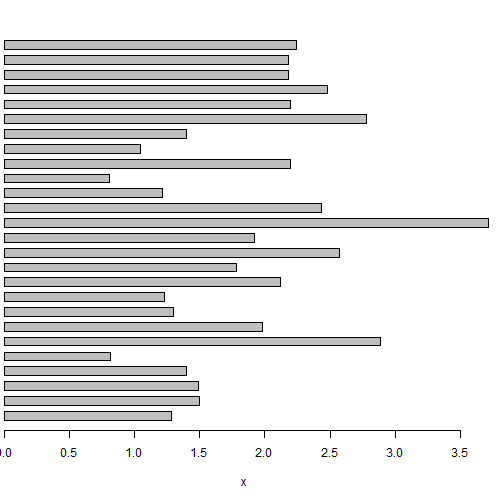However, it might be handy if, for instance, we wanted to create an array of `plotTree.barplot` plots - which we might do as follows:

``````X<-fastBM(tree,nsim=4,a=2,bounds=c(0,Inf))
par(mfrow=c(2,4))
colnames(X)<-paste("x",1:4,sep="")
``````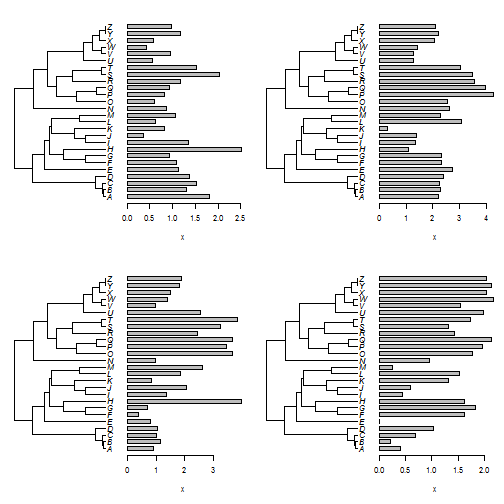Or, with the column names as axis labels:

``````par(mfrow=c(2,4))
args.barplot=list(xlab=name)),lapply(1:ncol(X),function(i,x) x[,i],x=X),
colnames(X),MoreArgs=list(tree=tree))
``````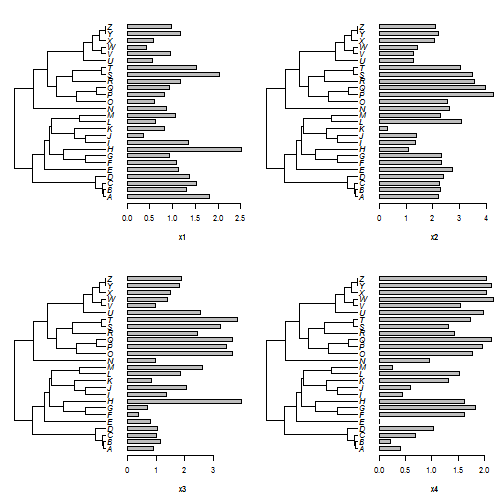Neat.

Finally, the other thing we could do with this is modify the amount of space we leave for the barplot & tree respectively. This could be particularly useful if our barplot contains a lot of information. For, here I'll use a 'stacked barplot' style for all four columns of `X`:

``````layout(matrix(c(1,2),1,2),widths=c(0.3,0.7))